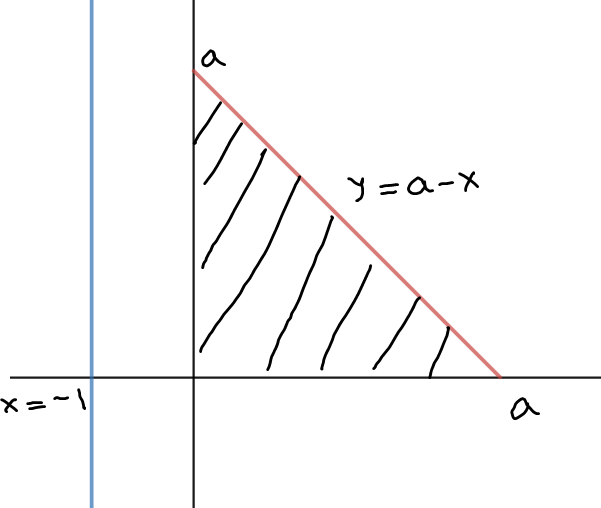# sketch the solid obtained by rotating the region underneath the graph of f over theinterval about the given axis, and calculate its volume using the Shell Method. f (x) = a − x with a > 0, [0, a], about x = −1

Question
1 views

sketch the solid obtained by rotating the region underneath the graph of f over the
interval about the given axis, and calculate its volume using the Shell Method. f (x) = a − x with a > 0, [0, a], about x = −1

check_circle

Step 1

We roughly graph the equations...

### Want to see the full answer?

See Solution

#### Want to see this answer and more?

Solutions are written by subject experts who are available 24/7. Questions are typically answered within 1 hour.*

See Solution
*Response times may vary by subject and question.
Tagged in